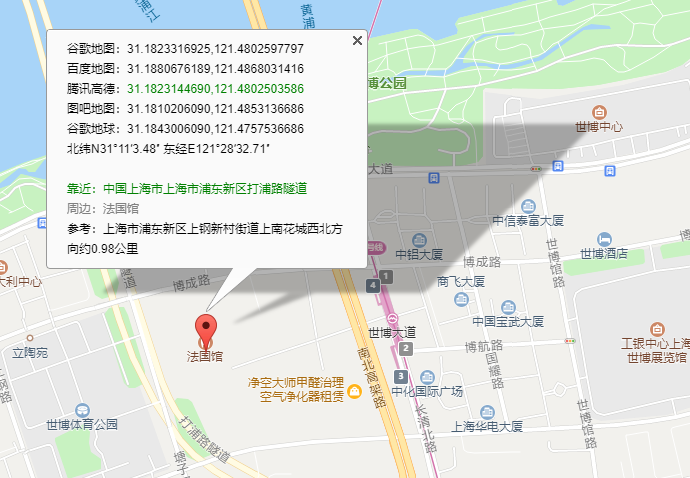# WGS84、WebMercator、GCJ02和BD09坐标系简介与转换

WGS84（GPS）

• 地心坐标系，空间直角坐标系，原点与地球质心重合，为GPS采用的坐标系，也是目前广泛使用的GPS全球卫星定位系统使用的坐标系。
• 通过GPS可以直接获取WGS84下的坐标(B，L，H)，B为纬度，L为经度，H为大地高即到WGS84椭球面的高度；
• 我国地图采用的是北京1954或西安1980坐标系下的高斯投影坐标(x,y)，也有采用北京1954或西安1980坐标系下的经纬度坐标(B,L)，高程一般为海拔高度；
• 世界大地坐标系是美国国防部制图局（Defence Mapping Agency， DMA）为统一世界大地坐标系统，实现全球测量标准的一致性，定义用于制图、大地、导航的坐标基准。
• 它包括标准地球坐标框架、用于处理原始观测数据的标准椭球参考面（即基准和参考椭球）和定义标准海平面的重力等势面（大地水准面）。
• GPS的测量结果与北京54或西安80坐标相差几十米到一百多米，随区域各异；
•  WGS 1984 的具体定义参数

GCS_WGS_1984
WKID: 4326 Authority: EPSG

Angular Unit: Degree (0.0174532925199433)
Prime Meridian: Greenwich (0.0)
Datum: D_WGS_1984
Spheroid: WGS_1984
Semimajor Axis: 6378137.0
Semiminor Axis: 6356752.314245179
Inverse Flattening: 298.257223563

WebMercator投影坐标系统，其基准面是 WGS1984

• Web Mercator 坐标系使用的投影方法不是严格意义的墨卡托投影，而是一个被 EPSG（European Petroleum Survey Group）称为伪墨卡托的投影方法，这个伪墨卡托投影方法的大名是 Popular Visualization Pseudo Mercator，PVPM。
• 谷歌地图（WGS_1984_Pseudo_mercator）、Virtual Earth、Bing Maps、百度地图、Mapabc、ArcGIS Online等采用Web Mercator或Spherical Mercator坐标系，天地图采用CGCS2000国家大地坐标系；
• 在投影过程中，将表示地球的参考椭球体近似的作为正球体处理（正球体半径 R = 椭球体半长轴 a）。这也是为什么在 ArcGIS 中我们经常看到这个坐标系叫 WGS 1984 Web Mercator (Auxiliary Sphere)。Auxiliary Sphere 就是在告知你，这个坐标在投影过程中，将椭球体近似为正球体做投影变换，虽然基准面是WGS 1984 椭球面。（Web Mercator与常规墨卡托投影的主要区别就是把地球模拟为球体而非椭球体）
• 尽管这个坐标系由于精度问题一度不被GIS专业人士接受，但最终 EPSG 还是给了 WKID:3857。

GCJ02又称火星坐标系，是由中国国家测绘局制定的地理坐标系统，是由WGS84加密后得到的坐标系。

BD09（百度GCJ02）百度坐标系，在GCJ02坐标系基础上再次加密。其中bd09ll表示百度经纬度坐标，bd09mc表示百度墨卡托米制坐标。

  BMapToWebMercatorTransform:function (lng,lat) {
console.log("百度地图坐标是（"+lng+","+lat+")");
//百度转GCJ-02
var x_pi = 3.14159265358979324 * 3000.0 / 180.0;
var x = lng - 0.0065;
var y = lat - 0.006;
var z = Math.sqrt(x * x + y * y) - 0.00002 * Math.sin(y * x_pi);
var theta = Math.atan2(y, x) - 0.000003 * Math.cos(x * x_pi);
var gg_lng = z * Math.cos(theta);
var gg_lat = z * Math.sin(theta);
//GCJ02转web墨卡托84
lng = gg_lng * Math.PI / 180 * earthRad;
var a = gg_lat * Math.PI / 180;
lat = earthRad / 2 * Math.log((1.0 + Math.sin(a)) / (1.0 - Math.sin(a)));
console.log("WebMercator坐标是（"+lng+","+lat+")");
},

WebMercator转百度坐标

WebMercatorToBMapTransform:function (lng,lat) {
console.log("WebMercator坐标是（"+lng+","+lat+")");
//Web墨卡托转GCJ02
lng = lng / 20037508.34 * 180;
var mmy = lat / 20037508.34 * 180;
lat = 180 / Math.PI * (2 * Math.atan(Math.exp(mmy * Math.PI / 180)) - Math.PI / 2);
//GCJ02转百度
var x_PI = 3.14159265358979324 * 3000.0 / 180.0;
var z = Math.sqrt(lng * lng + lat * lat) + 0.00002 * Math.sin(lat * x_PI);
var theta = Math.atan2(lat, lng) + 0.000003 * Math.cos(lng * x_PI);
lng = z * Math.cos(theta) + 0.0065;
lat = z * Math.sin(theta) + 0.006;
console.log("百度地图坐标是（"+lng+","+lat+")");
}

84转web墨卡托

function WGS84ToWebMercator(wx,wy){
var x = wx *20037508.34/180;
var y = Math.log(Math.tan((90+wy)*Math.PI/360))/(Math.PI/180);
y = y * 20037508.34/180;
return {x:x,y:y};
}

web墨卡托84

function WebMercatorToWGS84(cx,cy){
var x = cx/20037508.34*180;
var y = cy/20037508.34*180;
y = 180/Math.PI*(2*Math.atan(Math.exp(y*Math.PI/180))-Math.PI/2);
return {x:x,y:y};
}

各个坐标系的坐标查询posted @ 2019-05-15 21:16  昜木辰deブログ  阅读(...)  评论(...编辑  收藏